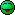# Center of Mass

## Homework Statement

Determine the position of the center of mass of a whole leg (a) when stretched out, and (b) when bent at 90°. Assume the person is 1.70 m tall.

## Homework Equations## The Attempt at a Solution

This is where I'm stuck...what's the mass? I doubt I can just use variable m because the mass is different parts of the leg. Also, when the problem says that the person is 1.7 m tall, should I use that for leg length and assume they meant leg length instead of height?

Related Introductory Physics Homework Help News on Phys.org
Is that all the information you are given? No diagram or anything? I think I would be led to believe that the point about the person being "1.70m tall" is some sort of red herring.

Yes, sadly that's all the information I'm given, not even a diagram.

gneill
Mentor
There's probably some standard body part model used in kinesiology and the like. No doubt there are mass models for sections of each limb, and I wouldn't be surprised if there's some sort of height index to scale the model.

Where does this problem come from?

It's from my physics homework.

I would guess that your professor assigned you this piece of work but forgot to reference where you could find the question in your textbook, maybe you'll find more information there